ZHCSDT9C June   2015  – June 2015

PRODUCTION DATA.

1. 特性
2. 应用
3. 说明
4. 修订历史记录
5. Device Comparison Table
6. Pin Configuration and Functions
7. Specifications
8. Parameter Measurement Information
9. Detailed Description
1. 9.1 Overview
2. 9.2 Functional Block Diagrams
3. 9.3 Feature Description
4. 9.4 Device Protection System
10. 10Application and Implementation
1. 10.1 Application Information
2. 10.2 Typical Applications
1. 10.2.1 Stereo BTL Application
1. 10.2.1.1 Design Requirements
2. 10.2.1.2 Detailed Design Procedures
2. 10.2.2 Application Curves
3. 10.2.3 Typical Application, Single Ended (1N) SE
4. 10.2.4 Typical Application, Differential (2N) PBTL
11. 11Power Supply Recommendations
1. 11.1 Power Supplies
2. 11.2 Powering Up
3. 11.3 Powering Down
12. 12Layout
13. 13器件和文档支持
14. 14机械、封装和可订购信息

• DDV|44

## 7 Specifications

### 7.1 Absolute Maximum Ratings

over operating free-air temperature range (unless otherwise noted) (1)
MINMAXUNIT
Supply voltageBST_X to GVDD_X(2)–0.350V
VDD to GND–0.313.2V
GVDD_X to GND(2)–0.313.2V
PVDD_X to GND(2)–0.350V
DVDD to GND–0.34.2V
AVDD to GND–0.38.5V
VBG to GND-0.34.2V
Interface pinsOUT_X to GND(2)–0.350V
BST_X to GND(2)–0.362.5V
OC_ADJ, M1, M2, OSC_IOP, OSC_IOM, FREQ_ADJ, C_START, to GND–0.34.2V
RESET, FAULT, CLIP_OTW, CLIP to GND–0.34.2V
INPUT_X to GND–0.37V
Continuous sink current, RESET, FAULT, CLIP_OTW, CLIP, RESET to GND9mA
TJOperating junction temperature range0150°C
TstgStorage temperature range –40150°C
(1) Stresses beyond those listed under Absolute Maximum Ratings may cause permanent damage to the device. These are stress ratings only, which do not imply functional operation of the device at these or any other conditions beyond those indicated under Recommended Operating Conditions. Exposure to absolute-maximum-rated conditions for extended periods may affect device reliability.
(2) These voltages represents the DC voltage + peak AC waveform measured at the terminal of the device in all conditions.

### 7.2 ESD Ratings

VALUEUNIT
VESDElectrostatic discharge Human body model (HBM), per ANSI/ESDA/JEDEC JS-001, all pins (1)±2000V
Charged device model (CDM), per JEDEC specification JESD22-C101, all pins(2)±500V
(1) JEDEC document JEP155 states that 500-V HBM allows safe manufacturing with a standard ESD control process.
(2) JEDEC document JEP157 states that 250-V CDM allows safe manufacturing with a standard ESD control process.

### 7.3 Recommended Operating Conditions

over operating free-air temperature range (unless otherwise noted)
MINTYPMAXUNIT
PVDD_xHalf-bridge supply DC supply voltage123638V
GVDD_xSupply for logic regulators and gate-drive circuitryDC supply voltage10.81213.2V
VDDDigital regulator supply voltageDC supply voltage10.81213.2V
RL(BTL)Load impedanceOutput filter inductance within recommended value range2.74Ω
RL(SE)1.53
RL(PBTL)1.62
LOUT(BTL)Output filter inductanceMinimum output inductance at IOC5μH
LOUT(SE)5
LOUT(PBTL)5
FPWMPWM frame rate selectable for AM interference avoidance; 1% Resistor toleranceNominal575600625kHz
AM1475500525
AM2430450470
R(FREQ_ADJ)PWM frame rate programming resistorNominal; Master mode9.91010.1
AM1; Master mode19.82020.2
AM2; Master mode29.73030.3
CPVDDPVDD close decoupling capacitors1.0μF
ROCOver-current programming resistorResistor tolerance = 5%2230
ROC(LATCHED)Over-current programming resistorResistor tolerance = 5%4764
V(FREQ_ADJ)Voltage on FREQ_ADJ pin for slave mode operationSlave mode3.3V
TJJunction temperature 0125°C

### 7.4 Thermal Information

THERMAL METRIC(1)TPA3251D2UNIT
DDV 44-PINS HTSSOP
JEDEC STANDARD 4 LAYER PCBFIXED 85°C HEATSINK TEMPERATURE(2)
RθJAJunction-to-ambient thermal resistance 50.72.5(2)°C/W
RθJC(top)Junction-to-case (top) thermal resistance 0.360.2
RθJBJunction-to-board thermal resistance 24.4n/a
ψJTJunction-to-top characterization parameter 0.190.5
ψJBJunction-to-board characterization parameter 24.2n/a
RθJC(bot)Junction-to-case (bottom) thermal resistance n/an/a
(1) For more information about traditional and new thermal metrics, see the IC Package Thermal Metrics application report, SPRA953.
(2) Thermal data are obtained with 85°C heat sink temperature using thermal compound with 0.7W/mK thermal conductivity and 2mil thickness. In this model heat sink temperature is considered to be the ambient temperature and only path for dissipation is to the heatsink.

### 7.5 Electrical Characteristics

PVDD_X = 36 V, GVDD_X = 12 V, VDD = 12 V, TC (Case temperature) = 75°C, fS = 600 kHz, unless otherwise specified.
PARAMETERTEST CONDITIONSMINTYPMAXUNIT
INTERNAL VOLTAGE REGULATOR AND CURRENT CONSUMPTION
DVDDVoltage regulator, only used as reference nodeVDD = 12 V33.33.6V
AVDD Voltage regulator, only used as reference nodeVDD = 12 V7.8V
IVDDVDD supply currentOperating, 50% duty cycle40mA
Idle, reset mode13
IGVDD_XGate-supply current per full-bridge50% duty cycle25mA
Reset mode3
IPVDD_XPVDD idle current per full bridge50% duty cycle with recommended output filter12.5mA
Reset mode, No switching1mA
RINInput resistance24
VIN Maximum input voltage swing 7V
IIN Maximum input current 1mA
G Inverting voltage GainVOUT/VIN20dB
OSCILLATOR
fOSC(IO+)Nominal, Master ModeFPWM × 63.453.63.75MHz
AM1, Master Mode2.8533.15
AM2, Master Mode2.582.72.82
VIH High level input voltage1.86V
VIL Low level input voltage1.45V
OUTPUT-STAGE MOSFETs
RDS(on)Drain-to-source resistance, low side (LS)TJ = 25°C, Includes metallization resistance,
GVDD = 12 V
60100
Drain-to-source resistance, high side (HS)60100
I/O PROTECTION
Vuvp,VDD,GVDDUndervoltage protection limit, GVDD_x and VDD9.5V
Vuvp,VDD, GVDD,hyst(1)0.6V
OTWOvertemperature warning, CLIP_OTW(1)115125135°C
OTWhyst(1)Temperature drop needed below OTW temperature for CLIP_OTW to be inactive after OTW event.25°C
OTE(1)Overtemperature error145155165°C
OTE-OTW(differential)(1)OTE-OTW differential30°C
OTEhyst(1)A reset needs to occur for FAULT to be released following an OTE event25°C
OLPCOverload protection counterfPWM = 600 kHz1.7ms
IOCOvercurrent limit protectionResistor – programmable, nominal peak current in 1Ω load, ROCP = 22 kΩ14A
IOC(LATCHED) Overcurrent limit protectionResistor – programmable, peak current in 1Ω load, ROCP = 47kΩ14A
IDCspkrDC Speaker Protection Current ThresholdBTL current imbalance threshold1.5A
IOCTOvercurrent response timeTime from switching transition to flip-state induced by overcurrent.150ns
IPDOutput pulldown current of each halfConnected when RESET is active to provide bootstrap charge. Not used in SE mode.3mA
STATIC DIGITAL SPECIFICATIONS
VIH High level input voltageM1, M2, OSC_IOP, OSC_IOM, RESET1.9V
VIL Low level input voltage0.8V
Ilkg Input leakage current100μA
OTW/SHUTDOWN (FAULT)
RINT_PUInternal pullup resistance, CLIP_OTW to DVDD, FAULT to DVDD202632
VOHHigh level output voltageInternal pullup resistor33.33.6V
VOL Low level output voltageIO = 4 mA200500mV
Device fanoutCLIP_OTW, FAULTNo external pullup30devices
(1) Specified by design.

### 7.6 Audio Characteristics (BTL)

PCB and system configuration are in accordance with recommended guidelines. Audio frequency = 1 kHz, PVDD_X = 36 V, GVDD_X = 12 V, RL = 4 Ω, fS = 600 kHz, ROC = 22 kΩ, TC = 75°C, Output Filter: LDEM = 10 μH, CDEM = 1 µF, mode = 00, AES17 + AUX-0025 measurement filters,unless otherwise noted.
PARAMETERTEST CONDITIONSMINTYPMAXUNIT
POPower output per channelRL = 3 Ω, 10% THD+N220W
RL = 4 Ω, 10% THD+N175
RL = 3 Ω, 1% THD+N175
RL = 4 Ω, 1% THD+N140
THD+NTotal harmonic distortion + noise1 W0.005%
VnOutput integrated noiseA-weighted, AES17 filter, Input Capacitor Grounded60μV
|VOS|Output offset voltage Inputs AC coupled to GND2060mV
SNRSignal-to-noise ratio(1)111dB
DNRDynamic range115dB
PidlePower dissipation due to Idle losses (IPVDD_X)PO = 0, 4 channels switching(2)1W
(1) SNR is calculated relative to 1% THD+N output level.
(2) Actual system idle losses also are affected by core losses of output inductors.

### 7.7 Audio Characteristics (SE)

PCB and system configuration are in accordance with recommended guidelines. Audio frequency = 1 kHz, PVDD_X = 36 V, GVDD_X = 12 V, RL = 2 Ω, fS = 600 kHz, ROC = 22 kΩ, TC = 75°C, Output Filter: LDEM = 15 μH, CDEM = 1 µF, MODE = 11, AES17 + AUX-0025 measurement filters, unless otherwise noted.
PARAMETERTEST CONDITIONSMINTYPMAXUNIT
POPower output per channelRL = 2 Ω, 10% THD+N84W
RL = 3 Ω, 10% THD+N60
RL = 4 Ω, 10% THD+N47
RL = 2 Ω, 1% THD+N67
RL = 3 Ω, 1% THD+N48
RL = 4 Ω, 1% THD+N37
THD+N Total harmonic distortion + noise 1 W0.015%
Vn Output integrated noise A-weighted, AES17 filter, Input Capacitor Grounded115μV
SNR Signal to noise ratio(1)A-weighted100dB
DNR Dynamic range A-weighted101dB
Pidle Power dissipation due to idle losses (IPVDD_X)PO = 0, 4 channels switching(2)0.5W
(1) SNR is calculated relative to 1% THD+N output level.
(2) Actual system idle losses are affected by core losses of output inductors.

### 7.8 Audio Characteristics (PBTL)

PCB and system configuration are in accordance with recommended guidelines. Audio frequency = 1 kHz, PVDD_X = 36 V, GVDD_X = 12 V, RL = 2 Ω, fS = 600 kHz, ROC = 22 kΩ, TC = 75°C, Output Filter: LDEM = 10 μH, CDEM = 1 µF, MODE = 10, AES17 + AUX-0025 measurement filters, unless otherwise noted.
PARAMETERTEST CONDITIONSMINTYPMAXUNIT
POPower output per channelRL = 2 Ω, 10% THD+N355W
RL = 3 Ω, 10% THD+N250
RL = 4 Ω, 10% THD+N195
RL = 2 Ω, 1% THD+N285
RL = 3 Ω, 1% THD+N200
RL = 4 Ω, 1% THD+N155
THD+N Total harmonic distortion + noise 1 W0.05%
Vn Output integrated noise A-weighted, AES17 filter, Input Capacitor Grounded62μV
SNR Signal to noise ratio(1)A-weighted111dB
DNR Dynamic range A-weighted111dB
Pidle Power dissipation due to idle losses (IPVDD_X)PO = 0, 4 channels switching(2)1W
(1) SNR is calculated relative to 1% THD+N output level.
(2) Actual system idle losses are affected by core losses of output inductors.

### 7.9 Typical Characteristics, BTL Configuration

All Measurements taken at audio frequency = 1 kHz, PVDD_X = 36 V, GVDD_X = 12 V, RL = 4 Ω, fS = 600 kHz, ROC = 22 kΩ, TC = 75°C, Output Filter: LDEM = 10 μH, CDEM = 1 µF, mode = 00, AES17 + AUX-0025 measurement filters,unless otherwise noted.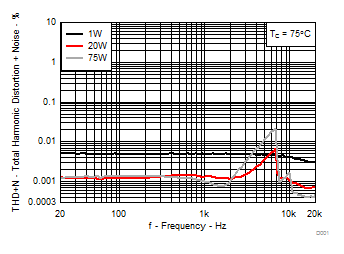RL = 4 Ω P = 1W, 20W, 75W TC = 75°C
Figure 1. Total Harmonic Distortion+Noise vs Frequency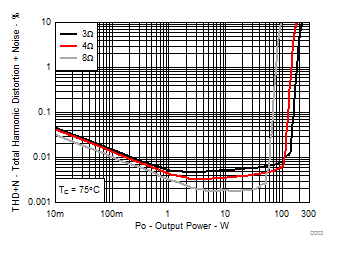RL = 3 Ω, 4 Ω, 8 Ω TC = 75°C
Figure 3. Total Harmonic Distortion + Noise vs Output Power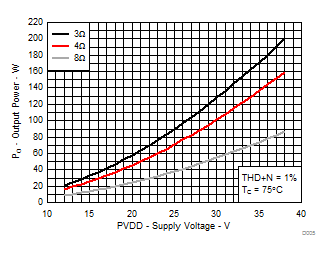RL = 3 Ω, 4 Ω, 8 Ω THD+N = 1% TC = 75°C
Figure 5. Output Power vs Supply Voltage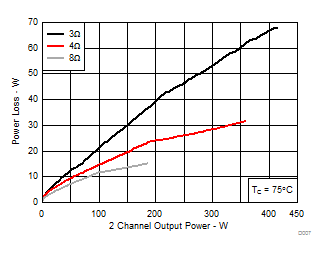RL = 3 Ω, 4 Ω, 8 Ω THD+N = 10% TC = 75°C
Figure 7. System Power Loss vs Output Power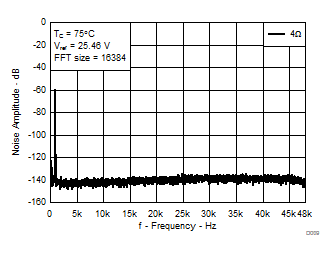4 Ω, VREF = 25.46 V (1% Output power) FFT = 16384 AUX-0025 filter, 80 kHz analyzer BW TC = 75°C
Figure 9. Noise Amplitude vs Frequency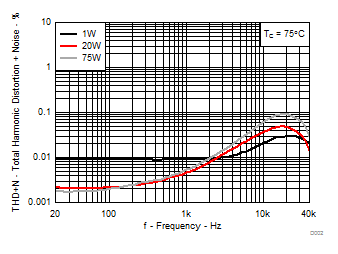RL = 4 Ω P = 1W, 20W, 75W TC = 75°C AUX-0025 filter, 80 kHz analyzer BW
Figure 2. Total Harmonic Distortion+Noise vs Frequency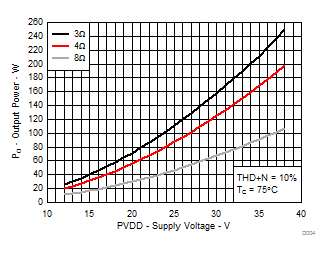RL = 3 Ω, 4 Ω, 8 Ω THD+N = 10% TC = 75°C
Figure 4. Output Power vs Supply Voltage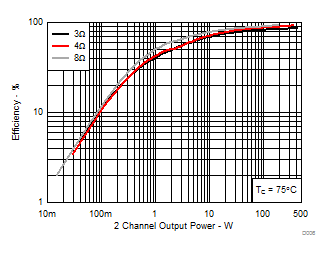RL = 3 Ω, 4 Ω, 8 Ω THD+N = 10% TC = 75°C
Figure 6. System Efficiency vs Output Power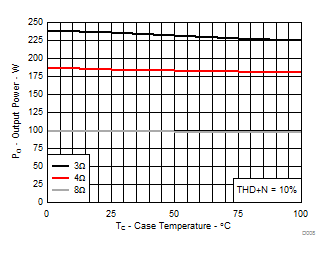RL = 3 Ω, 4 Ω, 8 Ω THD+N = 10% TC = 75°C
Figure 8. Output Power vs Case Temperature

### 7.10 Typical Characteristics, SE Configuration

All Measurements taken at audio frequency = 1 kHz, PVDD_X = 36 V, GVDD_X = 12 V, RL = 3 Ω, fS = 600 kHz, ROC = 22 kΩ, TC = 75°C, Output Filter: LDEM = 15 μH, CDEM = 680 nF, MODE = 11, AES17 + AUX-0025 measurement filters, unless otherwise noted.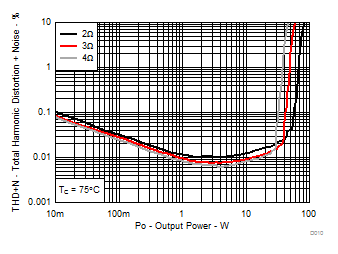RL = 2Ω, 3Ω, 4Ω TC = 75°C
Figure 10. Total Harmonic Distortion+Noise vs Output Power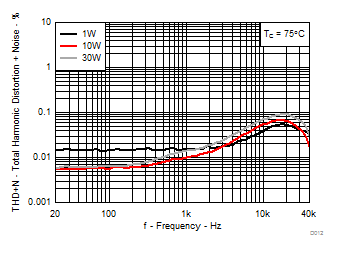RL = 3Ω P = 1W, 10W, 30W TC = 75°C AUX-0025 filter, 80 kHz analyzer BW
Figure 12. Total Harmonic Distortion+Noise vs Frequency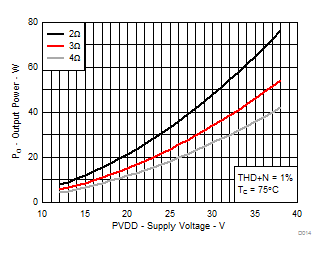RL = 2Ω, 3Ω, 4Ω THD+N = 1% TC = 75°C
Figure 14. Output Power vs Supply Voltage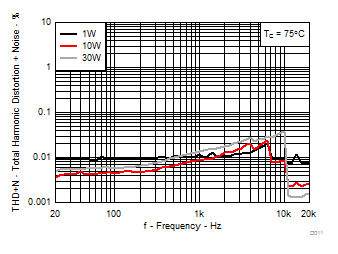RL = 3Ω P = 1W, 10W, 30W TC = 75°C
Figure 11. Total Harmonic Distortion+Noise vs Frequency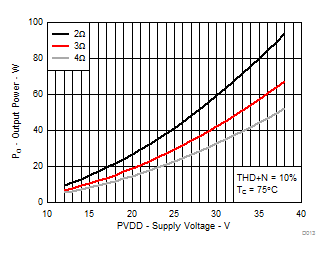RL = 2Ω, 3Ω, 4Ω THD+N = 10% TC = 75°C
Figure 13. Output Power vs Supply Voltage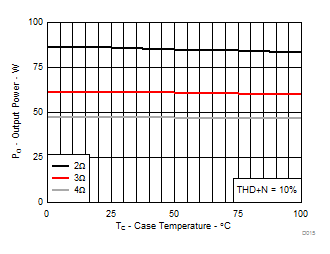RL = 2Ω, 3Ω, 4Ω THD+N = 10% TC = 75°C
Figure 15. Output Power vs Case Temperature

### 7.11 Typical Characteristics, PBTL Configuration

All Measurements taken at audio frequency = 1kHz, PVDD_X = 36V, GVDD_X = 12V, RL = 2Ω, fS = 600 kHz, ROC = 22kΩ, TC = 75°C, Output Filter: LDEM = 10μH, CDEM = 1 µF, MODE = 10, AES17 + AUX-0025 measurement filters, unless otherwise noted.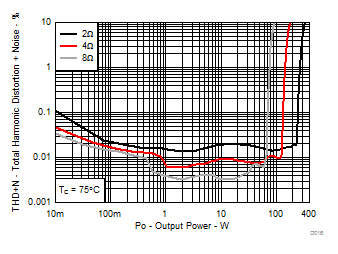RL = 2Ω, 4Ω, 8Ω TC = 75°C
Figure 16. Total Harmonic Distortion+Noise vs Output Power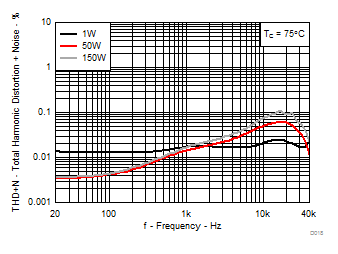RL = 2Ω P = 1W, 50W, 150W TC = 75°C AUX-0025 filter, 80 kHz analyzer BW
Figure 18. Total Harmonic Distortion+Noise vs Frequency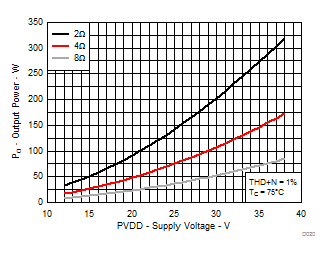RL = 2Ω, 4Ω, 8Ω THD+N = 1% TC = 75°C
Figure 20. Output Power vs Supply Voltage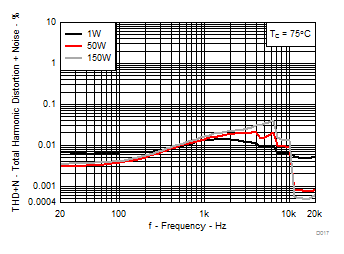RL = 2Ω P = 1W, 50W, 1.50W TC = 75°C
Figure 17. Total Harmonic Distortion+Noise vs Frequency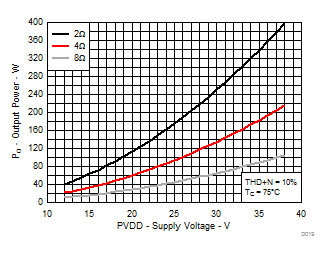RL = 2Ω, 4Ω, 8Ω THD+N = 10% TC = 75°C
Figure 19. Output Power vs Supply Voltage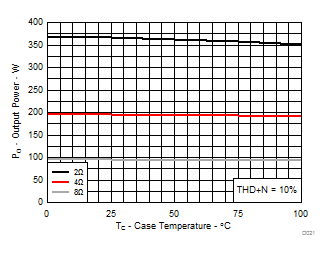RL = 2Ω, 4Ω, 8Ω THD+N = 10% TC = 75°C
Figure 21. Output Power vs Case Temperature